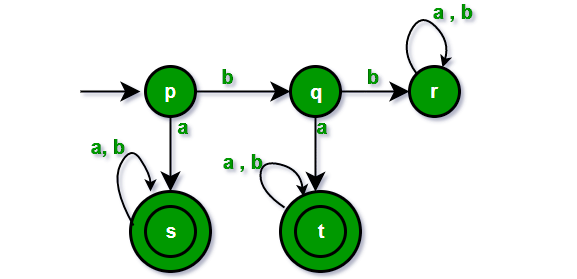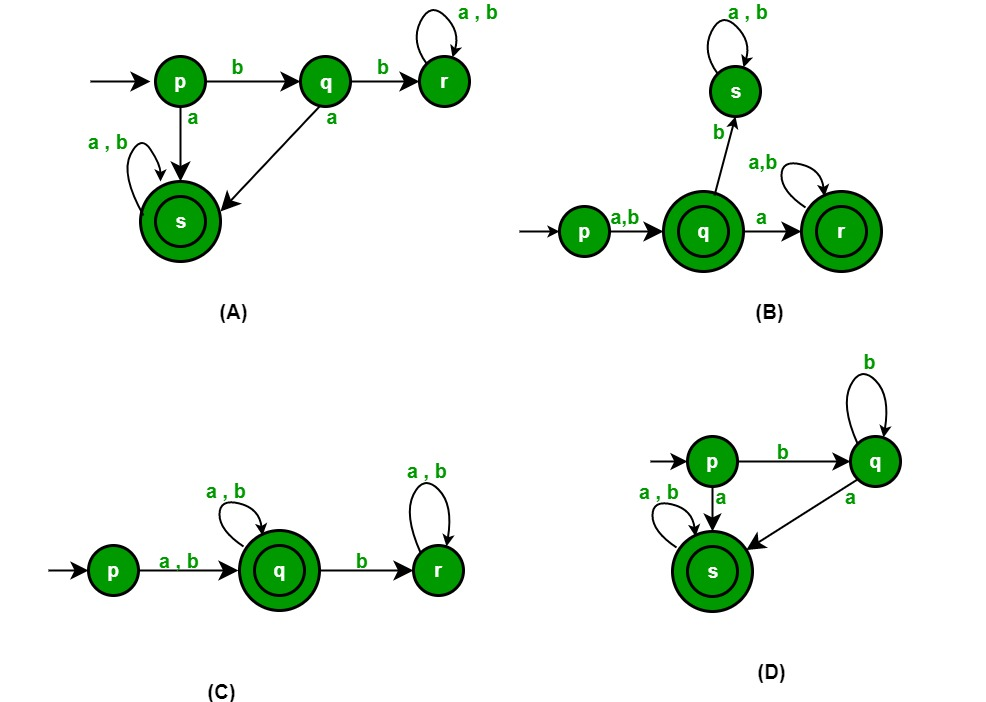# Automata Theory | Set 3

• Difficulty Level : Easy
• Last Updated : 13 Feb, 2018

Following questions have been asked in GATE CS 2011 exam.

1) The lexical analysis for a modern language such as Java needs the power of which one of the following machine models in a necessary and sufficient sense?
(A) Finite state automata
(B) Deterministic pushdown automata
(C) Non-deterministic pushdown automata
(D) Turing machine

Lexical analysis is the first step in compilation. In lexical analysis, program is divided into tokens. Lexical analyzers are typically based on finite state automata. Tokens can typically be expressed as different regular expressions:
An identifier is given by [a-zA-Z][a-zA-Z0-9]*
The keyword if is given by if.
Integers are given by [+-]?[0-9]+.

2) Which of the following pairs have DIFFERENT expressive power?
(A) Deterministic finite automata (DFA) and Non-Deterministic finite automata(NFA)
(B) Deterministic push down automata (DPDA) and Non-deterministic pushdown automata
(C) Deterministic single-tape Turing machine and Non-deterministic single-tape Turing Machine
(D) Single-tape Turing machine and multi-tape Turing machine

DPDA cannot handle languages or grammars with ambiguity, but NDPDA can handle languages with ambiguity and any context-free grammar.

3) A deterministic finite automation (DFA)D with alphabet ∑= {a,b} is given belowWhich of the following finite state machines is a valid minimal DFA which accepts the same language as D?